• ## c++ 构造函数详解

万次阅读 多人点赞 2019-05-31 17:20:58
c++构造函数详解。（构造函数的分类、拷贝构造函数


c++ 构造函数详解构造函数是干什么的构造函数的分类拷贝构造函数浅拷贝与深拷贝

c++ 构造函数详解
构造函数是干什么的
该类对象被创建的时候，编译系统对象分配内存空间，并自动调用该构造函数，由构造函数完成成员的初始化工作，故：构造函数的作用：初始化对象的数据成员
构造函数的分类
无参构造函数带默认值的构造函数有参（无默认值）的构造函数复制构造函数（拷贝构造函数）
一种特殊的构造函数，当对象之间复制时会自动调用拷贝构造函数若类中没有显示定义拷贝构造函数，则系统会自动生成默认拷贝构造函数
	#include <iostream>
using namespace std;

class Coordinate
{
public:
// 无参构造函数
// 如果创建一个类你没有写任何构造函数，则系统自动生成默认的构造函数，函数为空，什么都不干
// 如果自己显示定义了一个构造函数，则不会调用系统的构造函数
Coordinate()
{
c_x = 0;
c_y = 0;
}

// 一般构造函数
Coordinate(double x, double y):c_x(x), c_y(y){}   //列表初始化
// 一般构造函数可以有多个，创建对象时根据传入的参数不同调用不同的构造函数

Coordinate(const Coordinate& c)
{
// 复制对象c中的数据成员
c_x = c.c_x;
c_y = c.c_y;
}

// 等号运算符重载
Coordinate& operator= (const Coordinate& rhs)
{
// 首先检测等号右边的是否就是等号左边的对象本身，如果是，直接返回即可
if(this == &rhs)
return* this;
// 复制等号右边的成员到左边的对象中
this->c_x = rhs.c_x;
this->c_y = rhs.c_y;
return* this;
}

double get_x()
{
return c_x;
}

double get_y()
{
return c_y;
}

private:
double c_x;
double c_y;
};

int main()
{
// 调用无参构造函数，c1 = 0，c2 = 0
Coordinate c1, c2;
// 调用一般构造函数，调用显示定义构造函数
Coordinate c3(1.0, 2.0);
c1 = c3;    //将c3的值赋值给c1，调用"="重载
Coordinate c5(c2);
Coordinate c4 = c2;    // 调用浅拷贝函数，参数为c2
cout<<"c1 = "<<"("<<c1.get_x()<<", "<<c1.get_y()<<")"<<endl
<<"c2 = "<<"("<<c2.get_x()<<", "<<c2.get_y()<<")"<<endl
<<"c3 = "<<"("<<c3.get_x()<<", "<<c3.get_y()<<")"<<endl
<<"c4 = "<<"("<<c4.get_x()<<", "<<c4.get_y()<<")"<<endl
<<"c5 = "<<"("<<c5.get_x()<<", "<<c5.get_y()<<")"<<endl;
return 0;
}

	c1 = (1, 2)
c2 = (0, 0)
c3 = (1, 2)
c4 = (0, 0)
c5 = (0, 0)
请按任意键继续. . .

拷贝构造函数
拷贝构造函数是一种特殊的构造函数，具有单个形参，该形参（常用const修饰）是对该类型的引用。当定义一个新对象并用同一类型的对象都它进行初始化时，将显示使用拷贝构造函数，当该类型的对象传递给函数返回该类型的对象时，将隐式调用拷贝构造函数当类中有一个数据成员是指针时，或者有成员表示在构造函数中分配的其他资源，必须显示定义拷贝构造函数构造函数的使用情况
一个对象以值传递的方式传入函数体一个对象以值传递的方式从函数体返回一个对象需要通过另一个对象进行初始化
	#include <iostream>
using namespace std;

class Test
{
public:
// 构造函数
Test(int a):t_a(a){
cout<<"creat: "<<t_a<<endl;
}

// 拷贝构造函数
Test(const Test& T)
{
t_a = T.t_a;
cout<<"copy"<<endl;
}

// 析构函数
~Test()
{
cout<<"delete: "<<t_a<<endl;
}

// 显示函数
void show()
{
cout<<t_a<<endl;
}

private:
int t_a;
};

// 全局函数，传入的是对象
void fun(Test C)
{
cout<<"test"<<endl;
}

int main()
{
Test t(1);
// 函数中传入对象
fun(t);
return 0;
}

	creat: 1
copy
test
delete: 1
delete: 1
请按任意键继续. . .

浅拷贝与深拷贝
浅拷贝
所谓浅拷贝，指的是在对象复制时，只对对象中的数据成员进行简单的赋值，默认拷贝构造函数执行的也是浅拷贝。也就是增加了一个指针，指向原来已经存在的内存。 正常情况下，“浅拷贝”已经能很好的工作，但是一旦对象存在动态成员，浅拷贝就会出问题。让我们考虑下面一段代码：
	#include <iostream>
#include <assert.h>
using namespace std;

class Test
{
public:
Test(){
p = new int(10);
}

~Test(){
assert(p != NULL);     // assert()作用是如果他的条件返回错误，则终止程序执行
delete p;
}
private:
int x;
int y;
int* p;
};

int main()
{
Test t1;
Test t2(t1);    // 调用默认拷贝构造函数
return 0;
}

上述程序崩溃。在使用t1复制t2时，进行的是浅拷贝，只是将成员的值进行赋值。此时，t1.p = t2.p, 即两个指针指向了堆里的同一个空间。这样，析构函数会被调用两次，这就是错误出现的原因。此问题的解决方法是“深拷贝”。
深拷贝
深拷贝就是对于对象中的动态成员，并不只是简单的赋值，而是重新分配空间，即资源重新分配。上述代码处理如下：
	#include <iostream>
#include <assert.h>
using namespace std;

class Test
{
public:
Test(){
x = 0;
y = 0;
p = new int(10);
}

Test(const Test& t)
{
x = t.x;
y = t.y;
p = new int(10);
*p = *(t.p);
}

~Test(){
assert(p != NULL);     // assert()作用是如果他的条件返回错误，则终止程序执行
delete p;
}

int get_x(){return x;}
int get_y(){return y;}
private:
int x;
int y;
int* p;
};

int main()
{
Test t1;
Test t2(t1);    // 调用默认拷贝构造函数
cout<<"("<<t1.get_x()<<", "<<t1.get_y()<<")"<<endl
<<"("<<t2.get_x()<<", "<<t2.get_y()<<")"<<endl;
return 0;
}

(0, 0)
(0, 0)
请按任意键继续. . .


此时t1与t2的p各自指向一段内存空间，但他们指向的内容相同，这就是“深拷贝”。

展开全文c++ 浅拷贝 深拷贝
• ## MATLAB画函数图像

万次阅读 多人点赞 2019-04-19 15:56:34
1 画图基础 （1）一元一次函数 x=0:0.1:1; y=x; plot(x,y); %图像见下图1 图1 ...


1 画图基础
（1）一元一次函数
x=0:0.1:1;
y=x;
plot(x,y);   %图像见下图1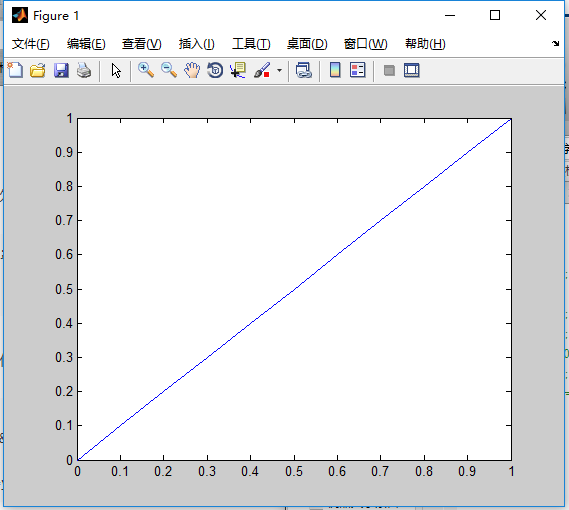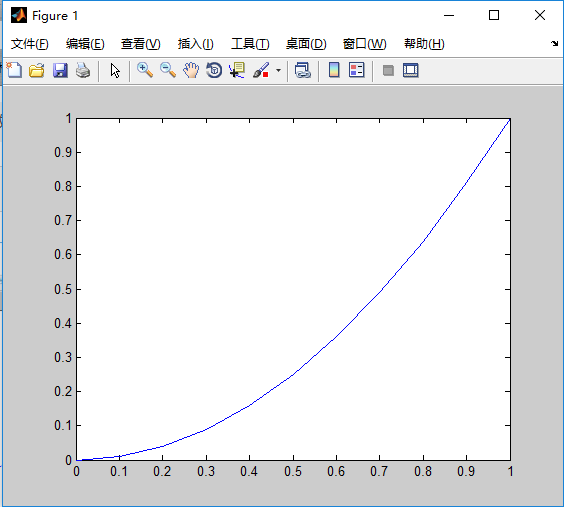图1                                                                                          图2
（2）一元多次函数
 x=0:0.1:1;
y=x.^2;
plot(x,y);    % 图像见图2
2 分段函数图像
x=0:0.1:2;
y=x.*(x>=0&x<=1)+(-(x-1).^2+1).*(x>1&x<=2);
plot(x,y);   %图像见图3
%组合函数y=y1.*(x定义域)+y2.*（x定义域）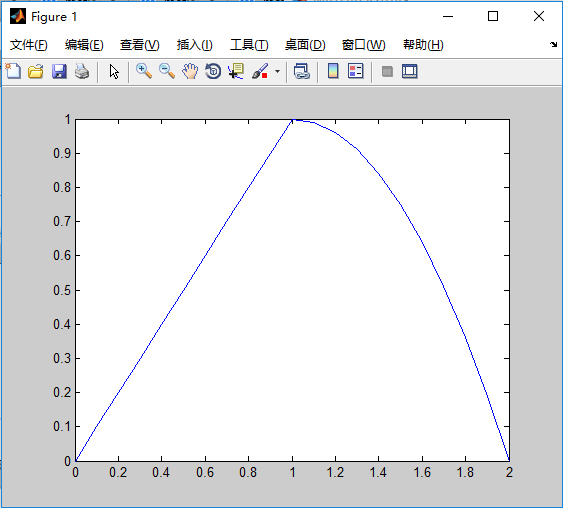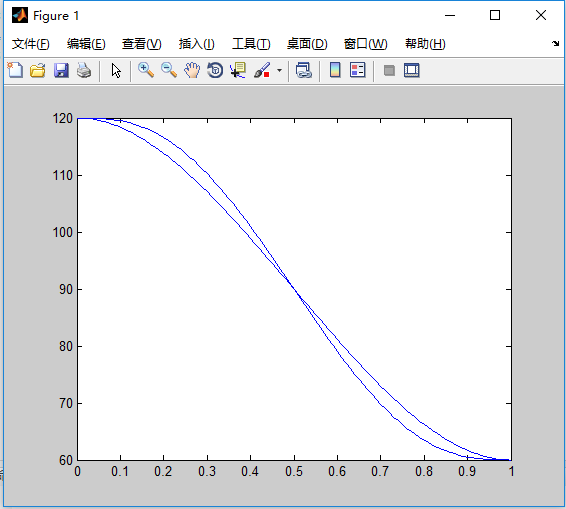图3                                                                                       图4
3 其他小技巧
（1）多条曲线画在同一个图像里
tip：使用 hold on 函数
t=[0:0.01:1];
q1=120-180*t.^2+120*t.^3;
q2=120-600*t.^3+900*t.^4-360*t.^5;
plot(t,q1);hold on;
plot(t,q2);
%图形见图4
（2）改变图像中线的颜色和线条形式（针对plot函数）
t=[0:0.01:1];
q1=120-180*t.^2+120*t.^3;
q2=120-600*t.^3+900*t.^4-360*t.^5;
plot(t,q1,'r');hold on;
plot(t,q2,'b');
%见图5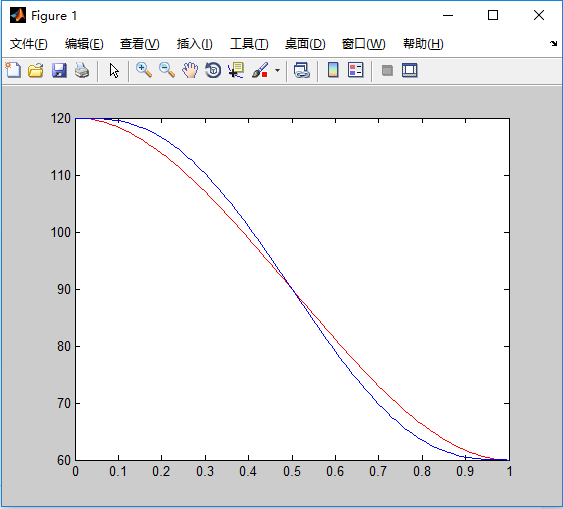图5
通过在plot（）函数括号里面增加特性来改变图线，常见的颜色和类型如图6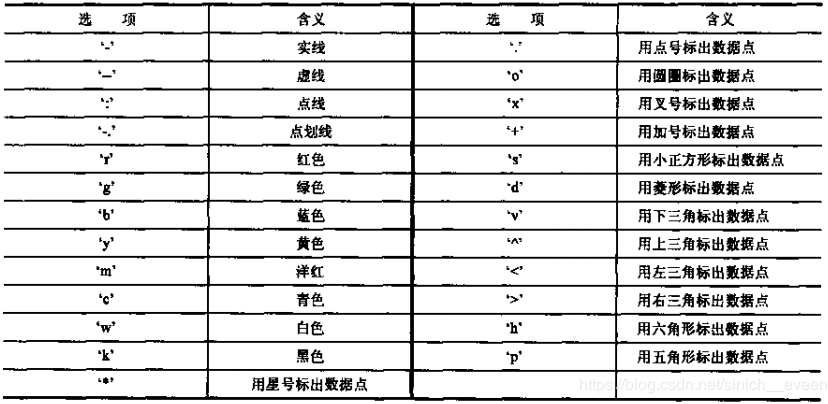图6
（3）增加图例
tip：利用l egend 函数
t=[0:0.01:1];
q1=120-180*t.^2+120*t.^3;
q2=120-600*t.^3+900*t.^4-360*t.^5;
plot(t,q1,'r'); hold on;
plot(t,q2,'b');
legend('a)函数图像','b)函数图像')  %要按函数的顺序来添加
%见图7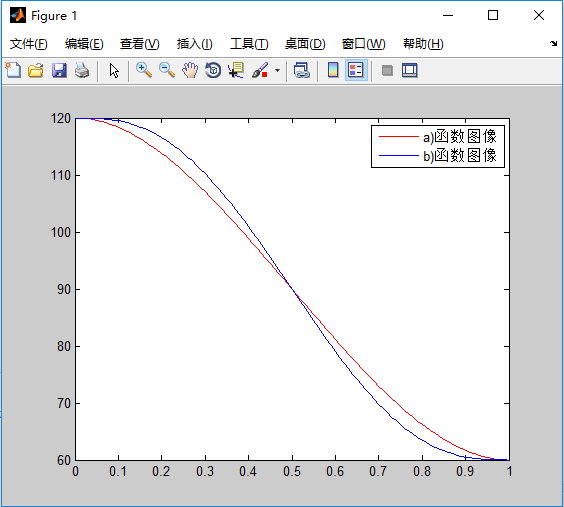图7

展开全文• 在matlab里.m文件分执行文件和函数文件 在c++中执行文件指：main函数 函数文件：其他所有需要用到的函数 在c++中，函数文件名没有特殊讲究，将文件添加到工程目录便能使用 对函数的要求有三点 函数的完整文件 输入...
在matlab里.m文件分执行文件和函数文件 在c++中执行文件指：main函数 函数文件：其他所有需要用到的函数
在c++中，函数文件名没有特殊讲究，将文件添加到工程目录便能使用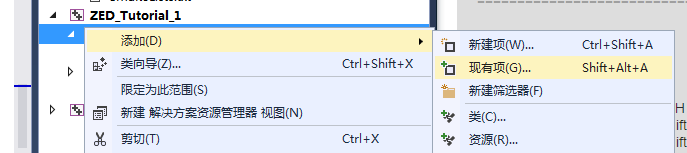对函数的要求有三点
函数的完整文件 输入参数的定义 函数声明加入头文件
1.函数的完整文件
#include <opencv2/opencv.hpp>
using namespace cv;

void cameracapture(Mat &frame, int mytime, int imageWidth,int imageHeight)
{
char c = 0;
VideoCapture capture(0);
capture.set(CV_CAP_PROP_FRAME_WIDTH, imageWidth*2);//宽度2560
capture.set(CV_CAP_PROP_FRAME_HEIGHT, imageHeight);//高度720

for (int i=0; i < mytime; i++)
{
capture >> frame;
}
}

函数的完整文件包括了函数需要的头文件
 #include <opencv2/opencv.hpp>
using namespace cv;

以及剩下的函数的程序段 这里解释一下加&和不加&的区别 Mat &frame 加&的变量传递的是变量地址，直白的理解为，加了后我在函数中对该变量修改后，会对我的主函数main中的对应变量进行修改。这里我的程序是打开相机，并把拍摄图像返回main函数，因此我需要随时根据拍摄修改我的main函数中frame的值。 int mytime, int imageWidth,int imageHeight这些则是传入值，在函数内进行修改后不影响main里面的值，因为这些值只传入函数，而不需要函数再传回主函数。 这里还有一点编程技巧 我们通过函数调用的方式进行运算，有两种方式得到运算结果 ①设置函数的返回值，return ②将传入值的地址（即传入值自身）交给函数，函数对其进行运算相当于直接对传入值进行运算。
2.输入参数的定义
我们在main中调用其他函数时，我们的输入参数需要提前定义
main ()
{
Mat frame;
int mytime = 10;
int imageWidth = 1280;
int imageHeight = 720;
cameracapture(frame, mytime, imageWidth, imageHeight);//注意这里和函数定义不同
………………
}

3.函数声明加入头文件
我们调用其他函数前必须先声明 将
 void cameracapture(Mat &frame, int mytime, int imageWidth,int imageHeight)

写入.h文件（头文件），写入头文件后也就告知了我们的项目，我们声明了，项目中是有该函数的定义的。为什么要用头文件？因为我们把我们用到的函数声明都写到一个.h文件里，下次再使用时我们直接#include XXX.h即可，没有必要再对用到的函数一个一个地声明。
完成上面的三步，我们自己的函数就制作好了，使用起来比较方便。 这里再扩展一下 我们在数组传入函数，传出函数时可能会面临着数组无法修改的问题，这里二郎给大家提供一个解决办法，不是最优，但是可行 main里面：
float key_data = { 0 };
my_f(img_cir_L, img_cir_R, key_data);

函数里面：
void my_f(Mat rectifyImageL, Mat rectifyImageR, float(&key_data))

头文件里面：
 void my_f(Mat rectifyImageL, Mat rectifyImageR, float(&key_data));

这样便能实现数组数据的传入和处理后结果的传递了
展开全文c++数组调用
• ## map函数

万次阅读 多人点赞 2019-04-21 22:10:44
map函数 map函数：在可迭代对象上映射函数 map函数在python2.0中，相关内置函数map在传入None作为函数参数时，会以类似方式把序列的元素配对起来。如果各个参数长度不等，那么map函数会用None补齐较短的序列（不是...
map函数
map函数：在可迭代对象上映射函数map函数在python2.0中，相关内置函数map在传入None作为函数参数时，会以类似方式把序列的元素配对起来。如果各个参数长度不等，那么map函数会用None补齐较短的序列（不是按照最短的序列长度截断），python3.0中不再支持在python3.0中，map会先输入一个函数参数，以及一个或多个序列参数，然后用从序列中取出的并行元素来调整，最后把结果收集起来并返回（map是一个值生成器，必须传入list从而一次性收集并显示其结果），返回的是迭代器
1. 函数语法：
map(function,iterable) function：函数 iterable：一个或者多个序列
2. map()会根据提供的函数对指定的序列做映射
第一个参数function()以参数序列中的每一个元素调用function函数，返回包含每次function函数返回值的新列表
>>> def square(x):
>>>     return x ** 2
>>> map(square,[1,2,3,4,5])
<map at 0xbd26f28>
>>> list(map(square,[1,2,3,4,5]))
[1, 4, 9, 16, 25]

>>> def add(x,y,z):
>>>     return x + y + z
>>> list1 = [1,2,3]
>>> list2 = [1,2,3,4]
>>> list3 = [1,2,3,4,5]
>>> print(list(res)) #1+1+1,2+2+2,3+3+3
[3, 6, 9]

展开全文python
• ## python中format函数

万次阅读 多人点赞 2019-05-14 12:07:45
python中format函数用于字符串的格式化 通过关键字 1. print('{name}在{option}'.format(name="谢某人"，option="写代码")) 结果：谢某人在写代码 通过位置 1. print('name={} path={}'.format('zhangsan', ...format
• ## python round函数

万次阅读 多人点赞 2019-01-24 13:00:59
如果 num_digits 大于 0，则四舍五入到指定的小数位。 如果 num_digits 等于 0，则四舍五入到最接近的整数。 如果 num_digits 小于 0，则在小数点左侧进行四舍五入。 示例 x=1.343671234 print ...
• ## 如何使用matlab实现分段函数

万次阅读 多人点赞 2018-10-09 20:39:13
实现如下的分段函数： m={t0≤t&amp;amp;amp;lt;1−t+21&amp;amp;amp;lt;t≤20.1其他 m=\left\{ \begin{array}{rcl} t &amp;amp;amp;amp; &amp;amp;amp;amp; {0 \leq t &amp;amp;amp;lt;1}...
• ## python求和函数sum()详解

万次阅读 多人点赞 2019-04-06 21:32:44
今天在学习的过程中，误用sum()函数，我又查了查python sum()函数才恍然大悟。 我本来想算几个Int值相加的和，本以为很简单的事情，结果却很悲伤，例： >>>sum = sum(1,2,3) #结果很明显出现问题报错 ...python python求和
• ## C++ 类(构造函数和析构函数)

万次阅读 多人点赞 2018-07-20 19:00:51
文章概述 构造函数和析构函数的由来？ 构造函数和析构函数的基本语法 C++编译器构造析构方案 PK 对象显示初始化方案 构造函数的分类以及调用 ...构造函数是一种特殊的成员函数,与其他函数不同,不需要用户调用它,而...
• 7-5 计算分段函数 (10 分) 本题目要求计算下列分段函数f(x)的值： 输入格式： 输入在一行中给出实数x。 输出格式： 在一行中按“f(x) = result”的格式输出，...f(234.0) = 234.0 7-5 计算分段函数 (10 分) ...
• 使用函数求余弦函数的近似值 ...cos (x) = x^0 / 0! - x^2 / 2! + x^4 / 4! - x^6 / 6! + ? 函数接口定义：funcos(eps,x ),其中用户传入的参数为eps和x；函数funcos应返回用给定公式计算出来，保留小数4位。 函数接...Python
• ## sort函数用法

万次阅读 多人点赞 2018-04-10 09:30:54
sort函数是一个非常强大的排序函数用法如下：//使用sort函数进行升序排序#include &lt;stdio.h&gt;#include &lt;algorithm&gt;//sort函数所在的函数库using namespace std;//sort所在的标准命名空间...
• ## c语言对数函数log的使用

万次阅读 多人点赞 2018-04-08 13:49:21
c语言log函数使用：   #include&lt;stdio.h&gt; #include&lt;math.h&gt; int main(){ printf("%f\n",log(10)); //以e为底的对数函数 printf("%f\n",log10(100)); //以...
• ## MATLAB 中的randn函数

万次阅读 多人点赞 2019-08-31 22:55:55
randn：产生均值为0，方差σ^2 = 1，标准差σ= 1的正态分布的随机数或矩阵的函数。 用法： Y = randn(n)：返回一个n*n的随机项的矩阵。如果n不是个数量，将返回错误信息。 Y = randn(m,n)或Y = randn([m n])：...randn
• 例子：N=[0 1 2 3 4 5 6 7 8 9 10 11 12 ];X=[0 1 2 3 1 0 -1 -2 -3 -4 -5 1 2];M=N';subplot(2,2,1),stem(N,X);subplot(2,2,2),stem(N);subplot(2,2,3),stem(M);显示如图：N是序列号 即从0到12号共13个数，注意必须...matlab
• 使用函数求素数和 prime§, 其中函数prime当用户传入参数p为素数时返回True，否则返回False. PrimeSum(m,n),函数PrimeSum返回区间[m, n]内所有素数的和。 题目保证用户传入的参数1<=m<n。 函数接口定义： 在...Python
• 使用函数统计指定数字的个数 本题要求实现一个统计整数中指定数字的个数的简单函数。 CountDigit(number,digit ) 其中number是整数，digit为[1, 9]区间内的整数。函数CountDigit应返回number中digit出现的次数。 ...Python
• ## matlab 冲激函数表示

万次阅读 2017-01-09 14:37:52
matlab中有一个dirc（）函数，用于δ函数的表示。 想画出冲激函数图像，可以利用如下代码：clc; clear all; x=0:200; %x轴范围 y=dirac(x-50); %x=50处有δ函数，即δ(x-50) y=1.5*sign(y); %改变幅度 plot(x,y); ...matlab
• 练习2-11 计算分段函数（10 分） 本题目要求计算下列分段函数f(x)的值： 注：可在头文件中包含math.h，并调用sqrt函数求平方根，调用pow函数求幂。 输入格式: 输入在一行中给出实数x。 输出格式: 在一...
• 通过本函数可以返回一个或一组服从“0~1”均匀分布的随机样本值。随机样本取值范围是[0,1)，不包括1。应用：在深度学习的Dropout正则化方法中，可以用于生成dropout随机向量（dl）， 例如（keep_...python
• ## C语言windows.h库的常用函数（三）

千次阅读 多人点赞 2019-09-07 21:57:00
SetCursorPos函数 用途 ...SetCursorPos函数拥有x和y两个整型参数，作为鼠标指针的坐标位置（其中0,0坐标对应屏幕左上角） 示例 #include<windows.h> int main(){ int a=0; while(a<1000...windows.h
• logsig函数可以将神经元的输入（范围是整个实数集）映射到区间（0,1）中。从形状上可以知道为何被称为S型函数。 使用语法： net.layers{i}.transferFcn = ‘logsig’; （2）tansig 函数 功能：双曲正切...神经网络 MATLAB 神经网络学习
• 本题要求实现一个计算Fibonacci数的简单函数，并利用其实现另一个函数,输出两正整数m和n（0<m<n≤100000）之间的所有Fibonacci数的数目。 所谓Fibonacci数列就是满足任一项数字是前两项的和（最开始两项均定义...Python
• 引言 本节主要是介绍神经网络中常见的激活函数-----...在信息科学中，由于其单增以及反函数单增等性质，常被用作神经网络的激活函数，将变量映射到0,1之间。-------------摘自《百度百科》 sigmod函数也叫作Log...
• 而在指针中，指针函数函数指针、指针函数数组、函数指针数组、指向函数指针数组的指针等等概念看着又绕又头疼。本问总结了一下以上一些概念以及用法，并给出例程深化理解。 1. 指针函数 指针函数就是返回指针值...C语言 指针数组
• C++函数指针、指针函数、返回值为函数指针的函数浅谈 引言 函数指针、指针函数是C中重要而容易混淆的概念，博主将通过两个实例来说明这两个截然不同的概念。 而返回值为函数指针的指针函数就更难理解了，放在文章的...C++
• 由于是二元函数，有两个因变量。偏导数表示分别对某一个导数求导，如偏x导数、偏y导数。 高阶偏导数 对偏导数继续求导。以二元函数的二阶偏导数为例，偏x导数有两个偏导数、偏y导数有两个偏导数。 定理：如果二元...高等数学 偏导数 全导数
• ## 指针函数和函数指针

万次阅读 多人点赞 2019-03-30 16:21:36
很多人因为搞不清这两个概念，干脆就避而远之，我刚接触C语言的时候对这两个概念也比较模糊，特别是当指针函数函数指针、函数指针变量、函数指针数组放在一块的时候，能把强迫症的人活活逼疯。 其实如果理解了这些...
• 之前提到的激活函数是以阈值0（界限值）为界的，小于等于0，输出0，否则，输出1。类似于这样的切换输出函数被称之为“阶跃函数”。因此，可以说感知机的激活函数为阶跃函数。那么，如果感知机使用其他函数作为激活...
• ## C语言中access/_access函数的使用

万次阅读 多人点赞 2019-09-02 18:29:41
在Linux下，access函数的声明在<unistd.h>文件中，声明如下： int access(const char *pathname, int mode); access函数用来判断指定的文件或目录是否存在(F_OK)，已存在的文件或目录是否有可读(R_OK)、可...access...